Speakable and Unspeakable in Special Relativity: the Ageing of the Twins in the Paradox | OMICS International
Journal of Physical Mathematics
All submissions of the EM system will be redirected to Online Manuscript Submission System. Authors are requested to submit articles directly to Online Manuscript Submission System of respective journal.

# Speakable and Unspeakable in Special Relativity: the Ageing of the Twins in the Paradox

Guerra V* and Abreu RD

Department of Physics, Instituto Superior Técnico, Technical University of Lisbon, Portugal

*Corresponding Author:
Guerra V
Department of Physics
Instituto Superior Técnico
Technical University of Lisbon
Portugal
Tel: 2354561314
E-mail: [email protected]

Received Date: February 22, 2017; Accepted Date: March 20, 2017; Published Date: March 22, 2017

Citation: Guerra V, Abreu RD (2017) Speakable and Unspeakable in Special Relativity: the Ageing of the Twins in the Paradox. J Phys Math 8:218. doi: 10.4172/2090-0902.1000218

Copyright: © 2017 Guerra V, et al. This is an open-access article distributed under the terms of the Creative Commons Attribution License, which permits unrestricted use, distribution, and reproduction in any medium, provided the original author and source are credited.

Visit for more related articles at Journal of Physical Mathematics

#### Abstract

In previous papers, we have presented a general formulation of special relativity, based on a weaker statement of the postulates. In this work, the paradigmatic example of the twin paradox is discussed in detail. Within the present formulation of special relativity, a “non-paradoxical” interpretation of the asymmetric ageing of the twins emerges. It is based exclusively on the rhythms of the clocks, which are not related by the standard textbook expressions and shall not be confused with clock time readings. Moreover, the current approach exposes the irrelevance of the acceleration of the returning twin in the discussion of the paradox.

#### Keywords

Special relativity; Lorentz transformations;Einstein speed

#### Introduction

In previous works we undertook a reflection on the foundations of special relativity [1-6] where we present a general formulation of special relativity and reconcile the ideas of Lorentz and Poincaré of the existence of a “preferred reference frame” and Einstein’s “equivalence of all inertial frames.” A comprehensive overview of the theory is given in our last paper,  hereafter denoted as I, where it is shown that it is not correct to speak about two philosophies, as they are different aspects of one and the same theory. Herein we extend our previous results and illustrate the power of the present formulation with a detailed discussion of the twin paradox.

The analysis of the “paradoxes” of a physical theory is an important matter, since it questions and assigns physical meaning to the statements of the theory. Following Feynman et al. , a paradox is a situation which gives one answer when analyzed one way, and a different answer when analyzed another way, so that we are left in somewhat of a quandary as to actually what should happen. Of course, in physics there are never any real paradoxes because there is only one correct answer; at least we believe that nature will act in only one way (and that is the right way, naturally). So in physics a paradox is only a confusion in our own understanding.

The famous twin paradox emerges in the standard interpretation of special relativity, which says that each twin ages slower than the other during any part of the to-and-fro traject, but when they meet one of them is older. This is of course not possible if “ageing slower” refers exclusively to rhythms of clocks along the trip and nothing else happens. Although the twin paradox is addressed and “solved” in any introductory course on special relativity, it was at the origin of more than 25,000 articles in the literature  since it was launched by Langevin in 1911 , and new publications arise regularly (the references given are therefore merely indicative [8,10-31]. Therefore, one can only suspect that “perhaps the last word on the twin paradox has yet to be said” .

In this paper, we argue that the key point to understand the twin paradox remains misinterpreted in its usual “solutions.” We start with a brief overview of the problem and then criticise the two main lines used in its standard “resolution:” the “simultaneity” and the “general relativity” arguments. In fact, despite giving the correct final answer, the usual explanations have a severe interpretation mistake, as they fail both to make the distinction between clock rhythms and clock time readings  and to recognise the indeterminacy of special relativity [4,6].

The structure of this paper is the following. In the next section we very briefly review some of the main findings from our former work [1-6], with emphasis on the relation of rhythms between two clocks in relative motion and its connection with the usual time dilation expressions. The twin paradox is then presented and examined in the subsequent sections. Section 3 presents a short historical overview of the problem. Critical reviews of the standard “solutions” using either only special relativity and no reference to acceleration or making use of general relativity and acceleration are given in sections 4 and 5, respectively. Section 6 contains the general analysis made in the context of the present formulation of special relativity. Finally, section 7 summarises our main findings.

#### Brief Overview of the Theory

In this section we very quickly review some results from I . We expected the reader to be familiar with that paper and call a special attention to its sections 5, 6 and 8, of particular relevance to the ensuing discussion.

#### T﻿he IST transformation, Lorentzian time and Einstein speed

Our formulation is based on the definition of the rest system – as the system where the one-way speed of light in vacuum is isotropic – and on the IST transformation (Inertial [32-34]–Synchronized [2,4,35-37] Tangherlini ). In the usual configuration where the axis of the rest frame S and a moving frame S′ are aligned, the origin of S′′ moves along the x-axis of S with speed v in the positive direction, and the reference event is the overlapping of the origins of both frames at time zero, the IST transformation is given by Guerra and Abreu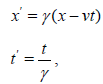(1)

with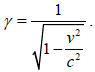(2)

It is important to note that the Lorentz transformation is readily obtained from the IST transformation by introducing the offset factor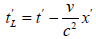(3)

and substituting t’ in (1) [2,4]. Therefore, any phenomenon that can be described by the Lorentz transformation can also be described by the IST transformation. We denote by Lorentzian clocks the clocks “synchronized” (i.e., “adjusted” or “set”) according to the Lorentz transformation, and by Lorentzian times the time readings exhibited by the Lorentzian clocks (3). Similarly, we denote by synchronized clocks the clocks adjusted according to the IST transformation and by synchronized times or simply “times” the time readings of the synchronized clocks.

The Lorentz transformation and the IST transformation can be associated with specific forms of clock “synchronization .” However, as stated in I, one simple – and yet critical – issue that has to be clarified before engaging any discussion on the interpretation of special relativity or of special relativity results is to state the difference between clock rhythms (or clock tick rates) and clock time readings (or time coordinates), as further discussed below. Contrary to time readings, clock rhythms do not depend on any particular form of “synchronization.” Surprisingly, the failure to make this basic distinction is at the origin of several misunderstandings surrounding the theory, including the discussion of the twin paradox.

The velocity addition formula can be obtained easily from (1) . If an object is moving at speed w in S, then its speed in the inertial frame S′, w'v , is simply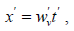(4)

which can be written in the form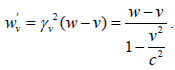(5)

It is also essential to distinguish speed (or “synchronized speed”) from Einstein speed [2,4,5]. Speed is defined as in the previous equations and can be calculated from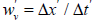. In turn, the Einstein speed is defined from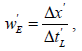(6)

i.e., its value is calculated with the difference of the time readings of Lorentizan clocks. Substituting (3) into (4), it is straightforward to show  that the Einstein velocity, w'E, measured in a frame moving with speed v (in S) of an object which has speed w, is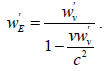(7)

Replacing w'v and using (5) this last expression can be rewritten as(8)

General expression for the rhythms of two clocks in relative motion

In prior work it was thoroughly debated that the indeterminacy of special relativity implies that just with Lorentzian clocks it is not possible to know in which inertial frame clocks are actually running slower . This result was derived more formally in section 8 of I, where the general expression for the rhythms of two clocks in relative motion was deduced. Due to its importance for the discussion of the twin paradox, we reproduce a big part of it here.

Consider two inertial frames, S′ and S′′, moving in the x direction respectively with speeds v and w in the rest system, S. Clock 1 is at the origin of and clock 2 is at the origin of S´´. The speed of clock 2 in S′is given by (5), the Einstein speed of clock 2 in S′ is given by (8). Let us further define the proper time, τ, in the usual way, as the time elapsed for one particular observer. Since the proper time is measured by a single clock, it is indeed associated with the clock rhythm and does not depend on the initial adjustment of distant clocks. The proper time of a clock in S′′ relates to the time elapsed in the rest system S by the time equation in (1), which can be written in the form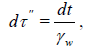(9)

where dt are the differential times marked by the different clocks in the rest system S that are co-punctual with clock 2 at each instant. The relation of the rhythm of a clock 1 in S′ with the rhythms of clocks in S is given by a similar expression, namely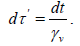(10)

Therefore, the relation of the proper times of clocks in S′′and in S´ is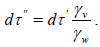(11)

Equation (11) establishes the relation of clock rhythms in two inertial frames. However, one final step is still missing. From (10) and (1) it directly follows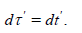(12)

Here, the equality is completely general and is valid whether or not dx′=0 as x′ does not appear in the second equation (1). In turn, as a consequence of (3), in general S′′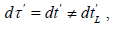(13)

although, if dx′=0, then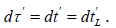Similarly, we can write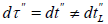, except when dx′′=0. Substituting (12) in (11), we get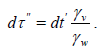(14)

The inverse relation is simply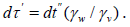As it is well-known from standard special relativity – and it is extremely easy to deduce from the Lorentz transformation – the proper time of clock 2 relates with the differential Lorentzian times through the Einstein speed,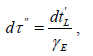(15)

Where γE is the Lorentz factor associated with the relative Einstein speed between both moving frames vE given by equation (8),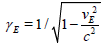. Contrary to (11), this expression corresponds to comparisons of time readings of Lorentzian clocks in S′ and does not correspond to a relation with the clock rhythms in S′.

Finally, by noting that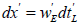and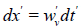, and using (13), equation (15) can be rewritten as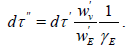(16)

This result confirms that Lorentzian clocks and Einstein speeds are not enough to determine in which frame clocks are running faster, since the relation between rhythms additionally involves the speed w'v .

The indeterminacy of special relativity  has thus been expressed in an alternative way. If the rest system is inaccessible, then we do not know the value of the one-way speed of light in one inertial frame, nor can we know the value of w'v in (16), and so we cannot know in which of two inertial frames clocks are ticking slower. This result, evidently, has profound implications in the analysis of the twin paradox.

#### Brief Overview

The twin paradox is one of the most famous paradoxes in physics. It emerges in the context of the standard interpretation of special relativity. Very briefly, consider twins Andrew and Bob . Andrew stays on the Earth, while Bob flies to a distant star and back. Within the standard interpretation os special relativity, from Andrew’s point of view Bob’s clock is running slower both on the outward and return parts of the trip. Therefore, Bob is younger than Andrew when they meet up again. The “paradox” part of the story comes from the following alternate reasoning. Within the standard interpretation of special relativity, in Bob’s frames it is Andrew’s clock which is running slower, and so it is Andrew who is younger than Bob when they meet up again. Of course this is self-contradictory, as Andrew cannot be younger and older than Bob.

About 10 years ago Peter Pesic wrote a very interesting review article on the twin paradox . In particular, he points out that, facing the paradox, Einstein addresses two arguments. The first one is the “simultaneity argument.” It uses only special relativity and does not attribute importance to acceleration other than to break the symmetry of the situation of both twins . This is indeed correct, as recognized by many physicists. For instance, Morin  states our time-dilation result holds only from the point of view of an inertial observer. The symmetry in the problem is broken by the acceleration. If both A and B are blindfolded, they can still tell who is doing the traveling, because B will feel the acceleration at the turnaround (...).

For the entire outward and return parts of the trip, B does observe A’s clock running slow, but enough strangeness occurs during the turning-around period to make A end up older. Note, however, that a discussion of acceleration is not required to quantitatively understand the paradox.

The somewhat uncomfortable but inescapable remark is that the change of inertial reference frames by Bob can be made in a very short time, in the limit even instantaneously. And it is this very short period, as compared to the trip duration, which is responsible for making Bob younger. Moreover, if Bob makes a longer trip and if he does the turn back exactly in the same way as before, he will return even younger than before. Thus, the effect of the very short turn-around period has to be higher now, in order to compensate for the longer period in which Bob sees Andrew ageing slower. The “solution” proposed by the standard interpretation of special relativity in this case is related to the “loss of simultaneity” exhibited by the time readings of Lorentzian clocks in different inertial frames [23,40]. If this is correct we have to acknowledge that “at the root of the twin paradox is the problem of synchronization of clocks .” However, this raises another major alarm. In fact, as repeated several times along in this work, physical results cannot depend on the way in which one decides to set his own clocks. This “solution” is analyzed in section 4.

In 1918 Einstein has advanced the second argument, the “general relativity argument” . It is now affirmed that we must consider general relativity in order to solve the paradox from the point of view of Bob. Since before and after the acceleration the argumentation of special relativity subsists, this comes back to attributing an important physical role to the acceleration. During the acceleration corresponding to the turn around period Bob would see Andrew age very quickly, because, according to the equivalence principle, Bob would see Andrew at a high gravitational potential. This second “solution” is discussed.

#### “Solution” without Acceleration

From the presentation of the broader view of special relativity proposed in I, in particular with the distinction between clock rhythms and clock time readings, together with the indeterminacy of special relativity, which does not allow to know in which inertial frame clocks are actually running slower, the meaningful solution to the paradox is already clear. The symmetry in the description of the outward trip between Andrew and Bob when we refer to Lorentzian times, repeated also for the return trip, does not correspond to a symmetry in the ageing (proper times) of the twins. Regarding proper times, we know that during the outward trip either Andrew or Bob is ageing slower and, without a reference to the rest system, we do not know which of them. And it may even happen that both are ageing at the same rhythm. The same occurs during the return trip. It is possible that it is always one of the twins who is ageing slower, both on the onward and on the return trip, or that one of the twins ages slower during the onward trip and the other during the return trip, or even that in one of the trips they are ageing at the same rate. However, we do know that when they meet it is Bob who is younger, and by which factor. Of course that all calculations can be made both with Lorentzian times and with synchronized times and from the point of view of each of the twins: all these calculations must give the same final result.

One quite convincing standard solution of the paradox using the “simultaneity argument” is obtained from the analysis of Minkowski diagrams.[39,10,20]. Let us assume that Bob travels along the x′ axis with Einstein speed of absolute value vE in relation to Andrew, both in the outward and on the return trips. There are various Lorentzian clocks along the x′ axis with photo cameras. At regular intervals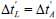in Andrew’s frame the cameras shoot. For each set of photos one and only one of the cameras has the photo of Bob. The situation is depicted in Figure 1. The universe line of Andrew is the ct′ axis (x′ =0), whereas the universe line of Bob is represented in red. Obviously, Andrew and Bob agree on the number N of photos taken during the all trip. It is straightforward to obtain the conversion of lengths in the diagram to the Lorentzian times in each frame [(one unit in the ct′′ axis)/(one unit in the ct′axis)=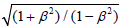, with β=v/c  and from simple trigonometry to get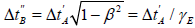. Notice that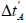refers to a comparison of the time readings of Lorentzian clocks in Andrew’s frame while '' corresponds to Bob’s proper time, so that this result is simply the symmetric description of time dilation obtained with Lorentzian clocks (cf. Figure 2 in I and its respective discussion). The total time elapsed for Andrew is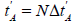, while for Bob the time lapse is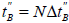, so that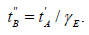(17)

Therefore, Bob is younger than Andrew when the twins meet. Expalined in Figure 1

Figure 1: Minkowski diagram for the twin paradox from Andrew's point of view (a) and from Bob's point of view (b).

To complete the analysis we have now to use the reverse reasoning, taking photos of Andrew from cameras in Bob’s frame, shot when the clocks associated with the cameras display the same Lorentzian time. Only one of each set of photos has Andrew’s photo. This new situation is represented in Figure 1b. There is not a single inertial frame associated with Bob, as the inertial frames for the onward and return trips are distinct. While Bob sees himself photographed at equal time intervals,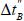in Figure 1a), Andrew seems himself being photographed regularly in the beginning of the trip,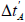in Figure 1b), then there is a time interval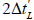(corresponding to the proximity of Bob with the star, the turn-around point) where no photo is taken, and then Andrew is again photographed regularly. As before, Andrew and Bob agree with the number of photos taken. Let d be the distance between the Earth and the star (in the Earth frame). The total time of the trip for Andrew is simply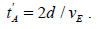(18)

The total time elapsed for Bob is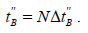(19)

Furthermore, in this case we have the reverse situation as compared to the previous figure concerning the intervalsand, with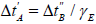, as derived from the Lorentz transformation or directly from the Minkowski diagram. Of courserefers to comparisons of Lorentzian times, whilecorresponds to Andrew proper times, so thatandare not the same as in the Figure 1a). It is also not difficult to show that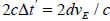. In other words, the time that passes for Andrew while he is not photographed, corresponding to Bob’s turn-around, is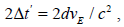(20)

i.e., the offset factor (3). The final calculation for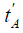then gives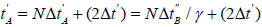(21)

from where, substituting (18–20), one recovers (17),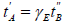, as it had to be. There is no paradox, and a careful calculation made only within standard special relativity leads to the right result, both from Andrew’s and from Bob’s points of view. In turn, failing to consider the factor Δt′ would lead to the paradoxical result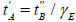m, contradictory with (17).

Despite the correctness of the result obtained with the Minkowski diagrams, the language used in the standard solution confuses “time” with Lorentzian times, making the interpretation somewhat awkward. In fact, without drawing the Minkowski diagrams and just using the Lorentz transformation, very few scientists would remember to add the offset factor 2dvE/c2 on a change of inertial frame corresponding to “Bob’s point of view” to get the final calculation right. This calculation error comes from a correct intuition: if the Loretzian time intervals would correspond to proper times, then one would not need to add, a bit artificially, some extra interval to the travel time of Andrew, which in addition depends on the distance, to correct for the instantaneous action of changing inertial frame. The need to add 2dvE/c2 to relate the Lorentzian times and compensate the different offset factors (3) of Bob’s two frames, together with the repeated remark that clock rhythms do not depend on the initial adjustment of the clocks, points the way to a clear and simple analysis of the twin paradox. Let us take one example to illustrate how does it work.

Consider the setup shown in Figure 3. Andrew and Bob are initially in a moving frame S′, which goes with speed v=0.4C in relation to the rest system S. A second moving frame S′′ goes with speed w=2v=0.8c. As in previous examples, all frames are equipped both with synchronized and with Lorentzian clocks. The same situation can then be described with both types of clocks. As the physical reality is not affected by the description chosen, one can use both types of clocks and both descriptions at the same time with no conflict at all. An apparent conflict only arises if one confuses Lorentzian clocks with synchronized ones.

Therefore, during the return trip one may be induced to think that Bob got younger by (5.76-4.34)ms=1.42 ms (Figure 2).

Figure 2: At t=10 ms Bob \jumps" to the moving frame S00, to the position of clock F, in order to catch up Andrew.

Figure 3: All times in the figures are expressed in milliseconds and rounded to two decimal places. Twins Andrew and Bob, initially in the moving frame S0, separate at t=0; Andrew (yellow dot) remains in frame S0 with clock D, while Bob (green dot) \jumps" to the rest system S to the location of clock A.

Just adding the differences of the Lorentzian time intervals we get the correct number and conclude that Bob is younger by (0.91+1.42) ms=2.33 ms, the same number obtained before, as it should to be: the physical reality does not depend on its description. Adding the Lorentzian time intervals provides the correct result, albeit an erroneous interpretation if we forget they are Lorentzian times and confuse them with clock rhythms.

Still within the reasoning of the standard interpretation of special relativity, Bob’s argumentation is much more striking and problematic. Bob considers himself to be at rest and so he expects to see Andrew ageing slower. He will compare the time readings of the Lorentzian clocks in his inertial frames (one frame during the onward trip, another one during the return trip) co-punctual with Andrew, with the time readings of Andrew’s clock. For the outward trip,

To keep the rules of the standard interpretation of special relativity, for the return trip we have to compare first the time readings of the Lorentzian clocks D and H in Figure 3, and then those of clocks D and F in Figure 4. Bob may then think that Andrew got even younger during the return trip, as for Andrew the difference in Lorentzian times is (18.33-9.17)ms=9.16 ms, whereas for Bob it is (22.67-11.33) ms=11.34 ms, so that one may think that Andrew got younger by (11.34-9.16)=2.18 ms. Therefore, not counting with any “strangeness” of the turn-around point, Bob may think that in total Andrew got younger by (0.83+2.18)ms=3.01 ms. However, Bob knows that at the turn-around point the Lorentzian clock H displays 11.33 ms Figure 3. However, his new Lorentzian clock F displays 16.67 ms! This difference of (16.67-11.33)ms=5.34 ms, which of course corresponds to the factor (20) resulting from the offset (3) of Lorentzian clocks, has to propagate to all clocks in S′′, as we are interested only in time-differences.

The above example confirms the validity of the “simultaneity argument,” in the sense that general relativity is not necessary for an explanation of the twin paradox and a correct calculation can be made within special relativity. It is not too complicate to perform all the formal calculations with Lorentzian and synchronized times from the expressions presented in section 2 and to arrive at the correct result (17), whatever the description and the twin point of view is adopted. Some of these calculations are presented in section 6.

#### Solution” with Acceleration

Let the total ageing in the round trip for Andrew be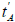, while for Bob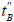. Any standard calculation made “from Andrew’s point of view” is trivial using time dilation and gives the result (17),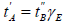, as shown in the previous section. It is the calculation made “from Bob’s point of view” which is more difficult. As we have just seen, the correct result can be obtained, e.g., from the analysis of Minkowski’s diagrams and only within the framework of special relativity. That being so, it should be clear that acceleration itself is not an essential ingredient in the solution of the paradox, other than to evince a lack of symmetry between the twins. However, it is also possible to use general relativity to explicitly account for the effect of acceleration. We take the standard argument from Tolman as presented by Grøn [27,42].

Consider a fixed coordinate non-inertial system with Bob. There are five different moments to take into account: 1) the acceleration of Bob up to speed v; 2) the travel up to the distant star at constant speed v; 3) the time required to reverse the speed at the distant star; 4) the back trip at constant speed –v; 5) the deceleration of Bob to meet Andrew. In order to keep the analysis as simple as possible, let us assume that all acceleration times in Bob’s frame are very short. According to general relativity we can replace all accelerations by gravitational fields and calculate the times processes 1) – 5) take in each frame. For the processes involving acceleration the relation of the time lapses in each frame is given by Tolman [42,27].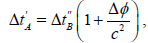(22)

where the gravitational potential difference Δφ is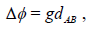(23)

dAB is the distance between Andrew and Bob and g is the proper acceleration of Bob.

For processes 1) and 5) Andrew and Bob are placed in the same location, so that dAB=0 As such, there is no difference in the gravitational potential and no difference in the ageing of the twins in these periods. In fact, the irrelevance of these periods is self-evident from the start, as Bob does not even have to accelerate nor to decelerate. Bob can simply fly past Andrew already at speed v at the beginning of the trip and compare his age with the one of Andrew when they cross, and then do exactly the same on the arrival. Next, during the onward and back trips, “Bob sees Andrew ageing slower.” As thoroughly debated along this paper, this assertion refers to the comparison of the displays of successive Lorentzian clocks in Bob’s frame co-punctual with Andrew, and not to clock rhythms. Thus, for processes 2) and 4) we have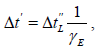(24)

where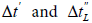are the proper time intervals for Andrew (as it they are measured with a single clock, carried by Andrew) and the Lorentzian time interval measured by different clocks in Bob’s frames in processes 2) and 4). Finally, for the turn around period 3), Bob is at a lower gravitational potential than Andrew, so that Bob’s clock runs slower according to (22), with dAB=d, the distance between Andrew and the distant star in Andrew’s frame. According to the standard argument, Bob sees Andrew moving freely upwards in a gravitational field until he stops, when he is at a distance d and then begins to fall down . Denoting by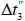the short duration of the acceleration leading to the velocity reversal in Bob’s frame, and noting that the proper acceleration is given by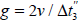, we have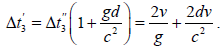(25)

Therefore, in the limit of short acceleration periods (→0 , g → ∞ ),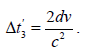(26)

Grøn  considers that the time interval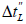in (24) corresponds to the actual ageing of Bob during processes 2) and 4), consistently with the assertion from the standard interpretation of special relativity that “for the entire outward and return parts of the trip, Bob does observe Andrews clock running slow.” Hence, it is claimed that Bob should calculate the time that passed for Andrew as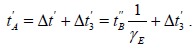(27)

This equation is the same as (21) and the additional factor (26) is precisely the same as the factor (20). Thus, the correct final result (17) directly follows and has now been obtained in an alternative way.

The standard interpretation of general relativity claims that while for Bob a very short period passes during the turn around period, during this time he sees Andrew ageing significantly because he is subject to an intense acceleration. Grøn  considers that special relativity leads to a paradox, as he assigns to the Lorentizan time interval in (24) the physical meaning of proper time. As such, he does not find a justification to the inclusion of the additional factor (26) within the context of special relativity and advocates a “general relativity solution.” He then presents two possible “solutions” to the question, an “elementary solution” and another from “using relativistic Lagrangian dynamics.” He acknowledges the difficulty of the former, as “it involves an assumption that is not obvious when the travelling twin stipulates the distance of his brother.” Although this is incorrect, it exposes the difficulties with the speech surrounding the standard interpretation of special relativity. A deeper analysis of this question will be given separately in a future publication.

Equation (27) contains a correct final result. But its customary interpretation is exceedingly misleading. For a start, it confuses proper times and Lorentzian clock time readings in expression (24). Then it misinterprets as well equation (22). In reality, the general relativity approach merely hides a procedure to compensate for the offset factors (3) associated with the Lorentzian clocks, as explicitly proven in the next section. Therefore, it does not bring any new physics and the obtained effect during the turn-around period described by (26) is not related to differences in proper times.

To finish this section, let us still note that for the discussion of the paradox the acceleration in the turn-around point can be eliminated in the same way as the accelerations in processes 1) and 5): a third twin can cross Bob at the turn around point, take note of Bob’s age when they cross, and then compare his own ageing since the meeting with Bob until he meets Andrew. He will age exactly the same as Bob in the limit Bob makes an instantaneous change in reference frame. This reinforces the indication that it is not the acceleration itself which is important, only the fact that there is one more inertial frame involved, with the corresponding modification of the clock rhythms.

#### General Solution

In this section we show that the broader view of special relativity proposed here demystifies the standard interpretations of the paradox both within the frameworks of special and of general relativity. It unveils, without ambiguity, that the additional factor (20) or (26) required to solve the twin paradox in either approach does not correspond to any physical effect on the ageing of either of the twins. It is simply a correction of the offset factors (3) resulting from Einstein’s procedure of “synchronization,” which are different for the two inertial frames associated with Bob in the roundtrip. This additional factor has no deeper meaning than to say that a person who suddenly advances his own clock by five minutes must then subtract five minutes to the time reading of his clock in order to know for how long he has been waiting. Let us then perform the calculations relevant to the twin paradox using the general expressions from section (2.2) and from the point of view of each of the twins. For simplicity, we again assume that Bob travels along the x′ axis with Einstein speed of absolute value vE in relation to Andrew, both in the outward and on the return trips.

The easiest calculation is the simple integration of equation (15), corresponding to using the standard expression “from Andrew’s point of view.” Keeping the same notation as in section 2.2, the actual ageing of the twins, given by the lapses in their proper times, is just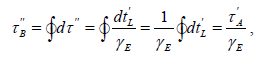(28)

where, notwithstanding (13),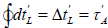. Indeed, although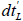is measured with different clocks from S′,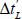in the roundtrip is measured with a single clock.

A second calculation can be made using again the standard relation, but “from Bob’s point of view.” This corresponds to the use of equation (15), interchanging the primed and double primed variables. In what follows the subscripts “+” and “–” refer to the outward and return trips, respectively. Note that in the onward and the return trips Bob uses different inertial frames. We have, successively,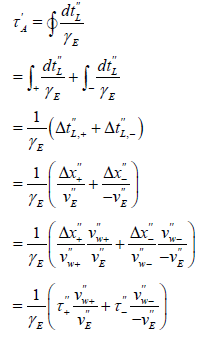(29)

where we have used (6). At this point we can show that Bob’s proper time lapses are the same on the onward and the return trips,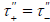. Take note that Bob’s ageing rhythm is different in each of the trips, except in the peculiar case in which Andrew is in the rest system. However, although the Einstein speed is the same the (synchronized) speed is not, which compensates for the difference in the rhythms. From (15),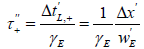(30)

and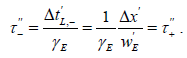(31)

Since,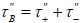, we have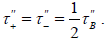(32)

We now need to eliminate the speeds of Andrew in Bob’s frame,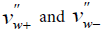, from (29). To do so, we note that, solving (8) for w,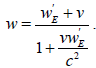(33)

In turn, exchanging the roles of v and w and the primed and double primed variables in (7),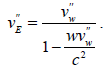(34)

Inverting for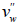and noting that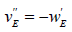, we get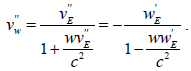(35)

Substituting (33) in this last expression,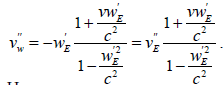(36)

Hence,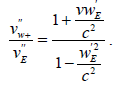(37)

Analogously, for the return trip we simply have to replace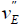by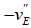, so that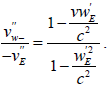(38)

Finally, substituting (32), (37) and (38) in (29),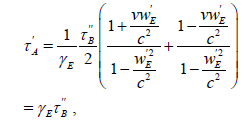(39)

so that we get once more the relation between the proper times of the twins (28). This somewhat dry but illuminating exercise proofs that the symmetric relations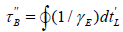(40)

and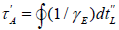(41)

are both valid and lead directly to the relation of rhythms,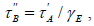(42)

with no need to include any additional factor. It further demonstrates that when it is said that “for the entire outward and return parts of the trip, B does observe A’s clock running slow” in the traditional symmetric account of time dilation, the expression “observing the clock running slow” is simply the comparison of the Lorentzian times of different clocks in B’s frame and has nothing to do with true clock rhythms. If it would correspond to the true ageing of the twins the paradox would remain, as it could not be simultaneously true that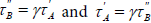. We would then have to add the factors (20) or (26) to Andrew’s proper time during Bob’s turn-around period when the calculation is made from Bob’s point of view, in order to compensate for the supposedly slower ageing of Andrew in the remaining of the trip. Incidentally, one should note that in the standard interpretation of relativity Bob needs to invoke an extremely fast ageing of Andrew, who can be light years apart, taking place exclusively during his own nearly instantaneous turn-around period. This additional factor has a much simpler nature, namely the offset factor (3), as we show next.

For simplicity, and without loss of generality, let us assume that Andrew remains in the rest system, S. Bob travels to the distant star and returns, with speed of absolute value v both in the onward and on the return trips. In this case, the absolute value of Bob’s Einstein speed in S coincides with the synchronized speed, vE= v and when Bob reaches the distant star the proper time elapsed for each of the twins is half of the total time elapsed in the complete round-trip. The ageing of Andrew until Bob arrives to the distant star can be calculated, e.g., as in (29)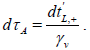(43)

Here,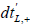refers to Lorentzian times displayed by different clocks in Bob’s frame, S′, co-punctual with Andrew, along the onward trip. Integrating along the onward trip,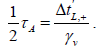(44)

Now, instead of proceeding as before, we can relate the Lorentzian time with the synchronized time and the proper time by (3) and (13), from where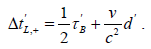(45)

Note that x′ in (3) is negative and d′=| x′| is the position of Andrew in Bob’s frame when he arrives to the star, i.e., d′/γv=d . Substituting in (44),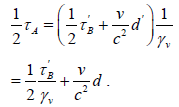(46)

Finally, noting that d = A /2 have, successively,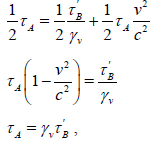(47)

which is again the true relation between the ageing of the twins.

The major and very consequential remark is to note that the second term in the r.h.s. of (??) is the additional factor (20) or (26). This expression is valid all along the one-way outward trip, in which Bob remains in the same inertial frame. It relates the proper times of both twins along the onward trip. It comes without saying that the additional factor has nothing to do neither with any strangeness at the turn-around point related to the “simultaneity argument” nor with acceleration, general relativity and the equivalence principle.

To summarize, for the particular case just considered the following relations are all valid all along the one-way onward trip,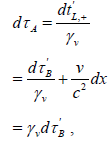(48)

and express the fact that Bob is ageing slower in this case. Understanding these three equalities clarifies the language and completely solves the twin paradox.

A straightforward analysis made with proper times and the IST transformation removes all difficulties surrounding the paradox at the onset. In particular, it is no longer said that each twin sees the other ageing slower during the length of the one-way trips. Instead, both twins agree that in each part of the trip one of them is ageing slower while the other is ageing faster (or are both ageing at the same rhythm). The simple addition of the proper times of the onward and back trips then gives directly the final result for both twins. There is no need to correct for any strangeness on the turn around period nor for any supposed effect of acceleration and equivalent gravitational fields. Specifically, there is no need to add any factor to Andrew’s age due to a change in inertial frame of Bob, whatever point of view is considered.

As a final remark, the general calculations can be made as well using (16) as a starting point, both as it is written and as well interchanging the roles of w and w and the primed and double primed variables, in an additional consistency check of the present formalism.

#### Conclusion

In previous papers we have proposed a general formulation of special relativity, where the postulates are formulated in a weaker form than in the traditional presentation, while keeping fully compatible with all experimental evidence [1-6]. The theory assumes the existence of (at least) one reference frame where the one-way speed of light in vacuum is isotropic and equal to c , denoted as the “rest system.” It was shown that the theory is undetermined, unless the one-way speed of light in one reference frame is measured . The somewhat evident but very important difference between “time readings of Lorentzian clocks” and proper times or clock rhythms is thoroughly discussed in I . It is noted that although the description of time dilation made with Lorentzian clocks is symmetrical for two inertial observers in relative motion, as it is well-known from the standard interpretation of special relativity, the reciprocal relation does not relate the clock rhythms . Actually, the time dilation relation between the clock rhythms of two inertial observers in relative motion is not symmetric. During each one-way trip one of the observers is actually ageing slower than the other, or it may even happen that both are ageing at the same rhythm. Furthermore, without reference to the rest system it is impossible to know which of them is actually ageing slower, in another way of stating the indeterminacy of special relativity .

Within this context, in this work we have discussed the twin paradox in detail, as an illustration of the power and simplicity of the general formulation of special relativity formerly presented. In a round-trip such as the one in the classical configuration of the twin paradox, it is the returning twin who is younger when both twin meets, despite the impossibility of knowing which of the twins is younger at each phase of the trip. The result is due to the cumulative effect of the clock rhythms along the complete journey.

The total ageing of each twin is calculated directly from the sum of their proper times on the onward and the return trips. Contrary to what happens in the standard interpretation of special relativity and of general relativity, there is no need to consider any additional ageing factor of the resting twin as seen by the moving twin to account for the change in reference frame or the acceleration (sections 4 and 5). As a matter of fact, the factor invoked by these standard interpretations was deduced from the offset between Lorentzian and synchronized clocks (section 5). It was thus demonstrated that this factor has nothing to do with any modification with the clock rhythms and with the ageing of the twins during the turn-around period. It is merely a correction to a peculiar way of giving the initial adjustment (a so-called “synchronization”) to the clocks. As a consequence, it becomes clear that acceleration does not play any role in the twin paradox other than telling which of the twins is returning back.

Finally, we would like to underline the following. The standard interpretation of special relativity pretends to assign a physical meaning of real ageing to assertions like “during the onward trip Bob sees Andrew ageing slower, Andrew himself also sees Bob ageing slower, but the change in inertial frames corrects this symmetry, as a result of the relativity of simultaneity, and makes it in the end that Bob is younger when the twins meet.” The standard interpretation of general relativity pretends to assign a physical meaning to sentences like “during the turn around period Bob sees Andrew ageing very quickly because he sees him under the effect of a gravitational field at a higher gravitational potential.” These are erroneous interpretations of correct mathematical results. One message we want to convey regarding the twin paradox is that such discourse is no longer tolerable and should become “unspeakable:” it was proven that “seeing the other twin ageing slower” is meaningless in this context and corresponds to the symmetric description arising from the comparison of the time readings of Lorentzian clocks (4 and 5), whose roots lie in the indeterminacy of special relativity . It does not correspond to the clock rhythms and to the ageing of the twins.

#### References

Select your language of interest to view the total content in your interested language

### Article Usage

• Total views: 2221
• [From(publication date):
March-2017 - Nov 18, 2019]
• Breakdown by view type
• HTML page views : 2084Can't read the image? click here to refresh